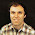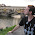## Friday, January 9, 2015

### The Electric Potential of a Rectangular Sheet of Charge

My idea of a great physics problem is one that is complicated enough so that it is not trivial, yet simple enough that it can be solved analytically. An example can be found in Sec. 6.3 of the 4th edition of Intermediate Physics for Medicine and Biology.
If one considers a rectangular sheet of charge lying in the xy plane of width 2c and length 2b, as shown in Fig. 6.10, it is possible to calculate exactly the E field along the z axis…. The result is

This is plotted in Fig. 6.11 for c = 1 m, b = 100 m. Close to the sheet (z much less than 1) the field is constant, as it is for an infinite sheet of charge. Far away compared to 1 m but close compared to 100 m, the field is proportional to 1/r as with a line charge. Far away compared to 100 m, the field is proportional to 1/r2, as from a point charge.
What I like most about this example is that you can take limits of the expression to illustrate the different cases. Russ Hobbie and I leave this as a task for the reader in Problem 8. It is not difficult. All you need is the value of the inverse tangent for a large argument (π/2), and its Taylor’s series, tan-1(x) = xx3/3 + . Often expressions like these will show simple behavior in two limits, when some variable is either very large or very small. But this example illustrates intuitive behavior in three limits. How lovely. I wish I could take credit for this example, but it was present in earlier editions of IPMB, on which Russ was the sole author. Nicely done, Russ.

Usually the electric potential, a scalar, is easier to calculate than is the electric field, a vector. This led me to wonder what electric potential is produced by this same rectangle of charge. I imagine the expression for the potential everywhere is extremely complicated, but I would be satisfied with an expression for the potential along the z axis, like in Eq. 6.10 for the electric field. We should be able to find the potential in one of two ways. We could either integrate the electric field along z, or solve for the potential directly by integrating 1/r over the entire sheet. I tried both ways, with no luck. I ground to a halt trying to integrate inverse tangent with a complicated argument. When solving directly, I was able to integrate over y successfully but then got stuck trying to integrate an inverse hyperbolic sine function with an argument that is a complicated function of x. So, I’m left with Eq. 6.10, an elegant expression for the electric field involving an inverse tangent, but no analytical expression for the electric potential.

I was concerned that I might be missing something obvious, so I checked my favorite references: Griffiths’ Introduction to Electrodynamics and Jackson’s infamous Classical Electrodynamics. Neither of these authors solve the problem, even for a square sheet.

As a last resort, I turn to you, dear readers. Does anyone out there—I always assume there is someone out there reading this—know of an analytic expression for the electric potential along the z axis caused by a rectangular sheet of charge, centered at the origin and oriented in the xy plane? If you do, please share it with me. (Warning: I suspect such an expression does not exist.) If you send me one, the first thing I plan to do is to differentiate it with respect to z, and see if I get Eq. 6.10.

This will be fun.

#### 6 comments:

1.When I read your post I first thought of these new spinal cord stimulater paddles. They are long epidural-catheter-looking objects with rectangular-shaped electrodes on them. (Boston Scientific's "Precision Spectra" is newer generation one) I imagined figuring the electric field experienced by the nerve--and then the activating function--as a superposition of your rectangular sheets.

To your question: can we take advantage of the superposition principle with a cleverly arranged array of the rectangles which gives an easy-to-compute potential, then infer the potential of the primitive rectangle from the potential of the array?

Thanks for posing the challenge! I will be on the hunt.

1.Frank Rattay has looked at the activating function under circular surface electrodes: Rattay, F. (1988). "Modeling the excitation of fibers under surface electrodes". IEEE Transactions on Biomedical Engineering 35 (3): 199–202.

You might find that paper helpful, if you have not already seen it.

2.For Friday fun, I couldn't find the closed form in Gradshteyn, but Mathematica's WolframAlpha took the following command:

indefinite integral of
arctangent((b c) /(z( c^2 + b^2 + z^2 )^(1/2) ) with respect to z

and spit out an analytic solution with a Laurent series expansion solution at infinity to boot.

Will have to chew on that for large and small b, c and z to see if it corresponds to the potentials we already know.

2.This comment has been removed by the author.

3.The problem is analogous to a problem in mathematical elasticity of a load on the surface of a three-dimensional half-space. The problem is outlined by mathematician A.E.H. Love in a 1929 paper. The solution you seek is not explicitly included in that paper, but is needed for the solution of the vertical displacement from a constant load over a rectangle, which I have solved. For your solution, I would try c*ln(r+b)+b*ln(r+c)-z*tan(bc/zr), with r=(c^2+b^2+z^2)^(1/2). Hope this helps.

1.Note a mistake, that should be an arctangent.